Refer to our Texas Go Math Grade 6 Answer Key Pdf to score good marks in the exams. Test yourself by practicing the problems from Texas Go Math Grade 6 Module 9 Answer Key Percents.

Write the equivalent fraction.

Question 1.Solution to this example is given below
$$\frac{9}{18}=\frac{9 \div 3}{18 \div 3}$$ = (Divide the numerator and denominator by the same)
= $$\frac{3}{6}$$ (number to find an equivalent fraction.)
Final solution = $$\frac{3}{6}$$

Question 2.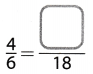Solution to this example is given below
$$\frac{4}{6}=\frac{4 \times 3}{6 \times 3}$$ = (Multiply the numerator and denominator by the same)
= $$\frac{12}{18}$$ (number to find an equivalent fraction.)
Final solution = $$\frac{12}{18}$$

Question 3.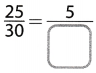Solution to this example is given below
$$\frac{25}{30}=\frac{25 \div 5}{30 \div 5}$$ = (Divide the numerator and denominator by the same)
= $$\frac{5}{6}$$ (number to find an equivalent fraction.)
Final solution = $$\frac{5}{6}$$

Question 4.Solution to this example is given below
$$\frac{12}{15}=\frac{12 \times 3}{15 \times 3}$$ = (Multiply the numerator and denominator by the same)
= $$\frac{36}{45}$$ (number to find an equivalent fraction.)
Final solution = $$\frac{36}{45}$$

Question 5.Solution to this example is given below
$$\frac{15}{24}=\frac{15 \div 3}{24 \div 3}$$ = (Divide the numerator and denominator by the same)
= $$\frac{5}{8}$$ (number to find an equivalent fraction.)
Final solution = $$\frac{5}{8}$$

Question 6.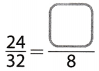Solution to this example is given below
$$\frac{24}{32}=\frac{24 \div 4}{32 \div 4}$$ = (Divide the numerator and denominator by the same)
= $$\frac{6}{8}$$ (number to find an equivalent fraction.)
Final solution = $$\frac{6}{8}$$

Question 7.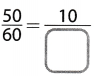Solution to this example is given below
$$\frac{50}{60}=\frac{50 \div 5}{60 \div 5}$$ = (Divide the numerator and denominator by the same)
= $$\frac{10}{12}$$ (number to find an equivalent fraction.)
Final solution = $$\frac{10}{12}$$

Question 8.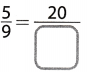Solution to this example is given below
$$\frac{5}{9}=\frac{5 \times 4}{9 \times 4}$$ = (Divide the numerator and denominator by the same)
= $$\frac{20}{36}$$ (number to find an equivalent fraction.)
Final solution = $$\frac{20}{36}$$

Multiply. Write each product in simplest form.

Question 9.
$$\frac{3}{8} \times \frac{4}{11}$$ = _____________
Write the problem as a single fraction
$$\frac{3 \times 4}{8 \times 11}$$
$$\frac{12}{88}$$ (Multiply numerators, Multiply denominators.)
$$\frac{12 \div 4}{88 \div 4}$$ (Simplify by dividing by the GCF.)
$$\frac{3}{22}$$ (Write the answer in simplest form.)

Question 10.
$$\frac{8}{15} \times \frac{5}{6}$$ = _____________
Write the problem as a single fraction
$$\frac{8 \times 5}{15 \times 6}$$
$$\frac{40}{90}$$ (Multiply numerators, Multiply denominators.)
$$\frac{40 \div 10}{90 \div 10}$$ (Simplify by dividing by the GCF.)
$$\frac{4}{9}$$ (Write the answer in simplest form.)

Question 11.
$$\frac{7}{12} \times \frac{3}{14}$$ = _____________
Write the problem as a single fraction
$$\frac{7 \times 3}{12 \times 14}$$
$$\frac{21}{168}$$ (Multiply numerators, Multiply denominators.)
$$\frac{21 \div 21}{168 \div 21}$$ (Simplify by dividing by the GCF.)
$$\frac{1}{8}$$ (Write the answer in simplest form.)

Question 12.
$$\frac{9}{20} \times \frac{4}{5}$$ = _____________
Write the problem as a single fraction
$$\frac{9 \times 4}{20 \times 5}$$
$$\frac{36}{100}$$ (Multiply numerators, Multiply denominators.)
$$\frac{36 \div 4}{100 \div 4}$$ (Simplify by dividing by the GCF.)
$$\frac{9}{25}$$ (Write the answer in simplest form.)

Question 13.
$$\frac{7}{10} \times \frac{20}{21}$$ = _____________
Given expression:
$$\frac{7}{10} \times \frac{20}{21}$$

Simplify, dividing out the common factor:
= $$\frac{1}{1} \times \frac{2}{3}$$
Evaluate:
= $$\frac{2}{3}$$
$$\frac{7}{10} \times \frac{20}{21}$$ = $$\frac{2}{3}$$

Question 14.
$$\frac{8}{18} \times \frac{9}{20}$$ = _____________
Write the problem as a single fraction
$$\frac{8 \times 9}{18 \times 20}$$
$$\frac{72}{360}$$ (Multiply numerators. Multiply denominators.)
$$\frac{72 \div 72}{360 \div 72}$$ (Simplify by dividing by the GCF.)
$$\frac{1}{5}$$ (Write the answer in simplest form.)

Multiply.

Question 15.
20 × 0.25 ___________
Solution to this example is given belowQuestion 16.
0.3 × 16.99 ________
Solution to this example is given below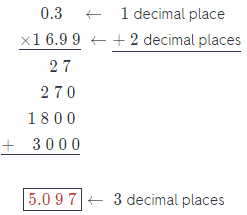Question 17.
0.2 × 75 __________
Solution to this example is given below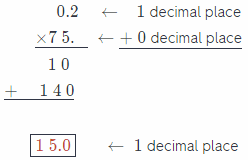Question 18.
5.5 × 1.1 __________
Solution to this example is given belowQuestion 19.
11.99 × 0.8 ________
Solution to this example is given below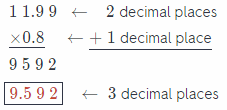Question 20.
7.25 × 0.5 _________
Solution to this example is given belowQuestion 21.
4 × 0.75 ___________
Solution to this example is given below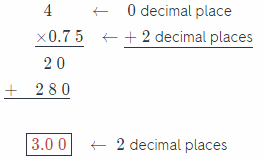Question 22.
0.15 × 12.50 _________
Solution to this example is given below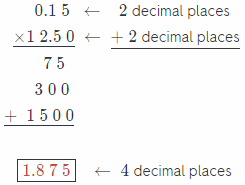Question 23.
6.5 × 0.7 __________
Solution to this example is given belowVisualize Vocabulary

Use the ✓ words to complete the graphic. You may put more than one word in each box.Understand Vocabulary

Match the term on the left to the correct expression on the right.